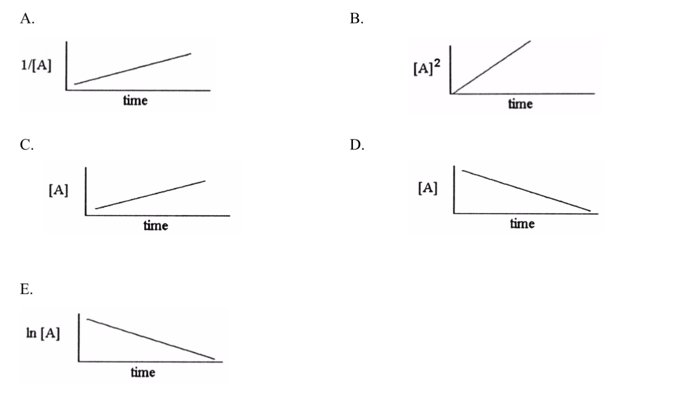# Problem: Given the unit of rate constant ( k ) as mol / (L•s), which one of the following graphs shows the correct relationship between concentration of reactant A and time for the reaction?

###### FREE Expert Solution
89% (426 ratings)
###### Problem Details

Given the unit of rate constant ( ) as mol / (L•s), which one of the following graphs shows the correct relationship between concentration of reactant A and time for the reaction?What scientific concept do you need to know in order to solve this problem?

Our tutors have indicated that to solve this problem you will need to apply the Identifying Reaction Order concept. If you need more Identifying Reaction Order practice, you can also practice Identifying Reaction Order practice problems.

What is the difficulty of this problem?

Our tutors rated the difficulty ofGiven the unit of rate constant ( k ) as mol / (L•s), which ...as medium difficulty.

How long does this problem take to solve?

Our expert Chemistry tutor, Dasha took 3 minutes and 20 seconds to solve this problem. You can follow their steps in the video explanation above.

What professor is this problem relevant for?

Based on our data, we think this problem is relevant for Professor Burnes' class at UNCG.